# Absolute value function

Jump to: navigation, search
This article is about a particular function from a subset of the real numbers to the real numbers. Information about the function, including its domain, range, and key data relating to graphing, differentiation, and integration, is presented in the article.
View a complete list of particular functions on this wiki

## Definition

The absolute value function, denoted as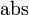$\operatorname{abs}$ or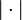$| \cdot |$, is defined as the function that sends a real number to itself if it is nonnegative and to its negative if it is negative.

The absolute value of$x$ is denoted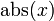$\operatorname{abs}(x)$ or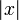$|x|$. It can be defined in many equivalent ways.

### As a piecewise linear function

The function is a piecewise linear function with the following piecewise definition:$|x| := \left\lbrace \begin{array}{rl}x, & x \ge 0 \\ -x, & x < 0 \\\end{array} \right.$

### As a max of two functions

The absolute value function can be defined as: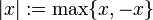$|x| := \max \{ x, -x \}$

In other words, it is obtained by applying the pointwise maximum to the following two functions: the identity function and the negative of the identity function.